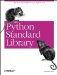# The pdb Module

The pdb module is the standard Python debugger. It is based on the bdb debugger framework.

You can run the debugger from the command line (type n [or next] to go to the next line and help to get a list of available commands):

```\$ pdb.py hello.py
> hello.py(0)?()
(Pdb) n
> hello.py()
(Pdb) n
hello again, and welcome to the show
--Return--
> hello.py(1)?()->None
(Pdb)```

Example 11-2 shows how to start the debugger from inside a program.

Example 11-2. Using the pdb Module

```File: pdb-example-1.py

import pdb

def test(n):
j = 0
for i in range(n):
j = j + i
return n

db = pdb.Pdb()
db.runcall(test, 1)

> pdb-example-1.py(3)test()
-> def test(n):
(Pdb) s
> pdb-example-1.py(4)test()
-> j = 0
(Pdb) s
> pdb-example-1.py(5)test()
-> for i in range(n):
...```Python Standard Library (Nutshell Handbooks) with
ISBN: 0596000960
EAN: 2147483647
Year: 2000
Pages: 252
Authors: Fredrik Lundh